NavBar

Modeling

# Computational Modeling of Conjugation

## Markov Modeling

### Introduction

To effectively evaluate the relationship between biocontainment and baseline conjugation occurring, Michigan State iGEM looked to computational strategies to analyze crucial pathways of plasmid transfer. The markov model, in particular, is useful for targeting the different mechanisms of plasmid transfer with the layers Synterception provides.

### Approach

The approach began with a Markov Model. A Markov Model comprises several transition states ruled by the probability of a change of states over time. Here, the Horizontal Gene Transfer Baseline Assay is modeled in terms of probability of susceptibility, conjugation, transformation. Above, Baseline Markov Model (BMM) uses adjustable variables alpha, beta, gamma, and delta. The simulation updates transition states based on the probabilities indicated on the slider (above the graph). The BMM provides data on outcomes of baseline conjugative behavior based on 4 simple rates. The end result represents a steady state, in which change is no longer observed. Transition states can be visualized as: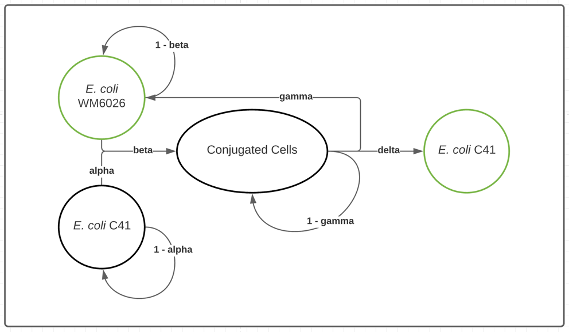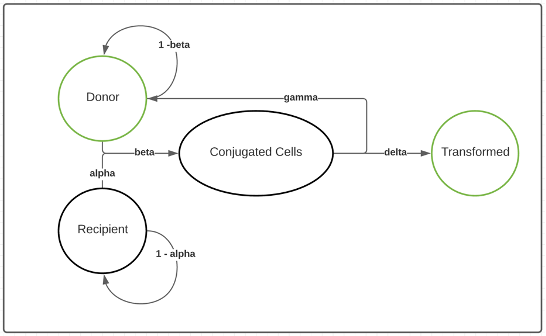### Results

The BMM produces plots such as: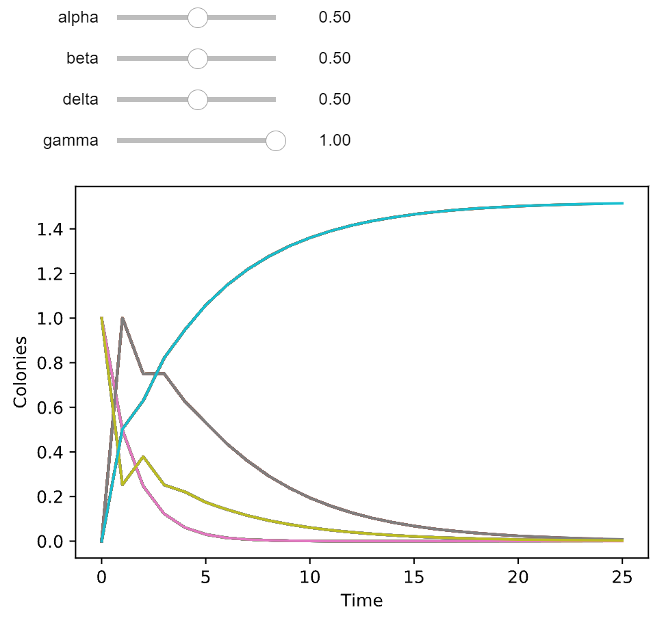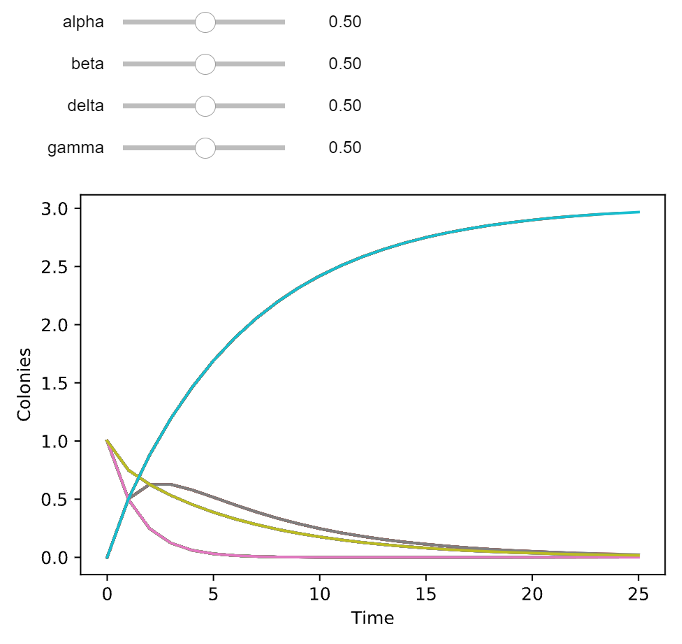Above, the plot is a result of a Python script initialized with two cells: one donor (pink) and one recipient (green). While the program calculates the states over time, the occurrences in conjugated cells (brown) and transconjugants (blue) change accordingly. As a tool for simulating the steady states for baseline conjugation occurring (respective to the Conjugative Baseline Assay), the slider pictured above the plot is adjustable in the program. The applied values on the slider correspond to the probability of transitioning cell states. Described below, the probabilities are defined by the mathematical and biological possibilities of transformation.

The probabilities are tabulated as a matrix seen below: Probability Matrix, P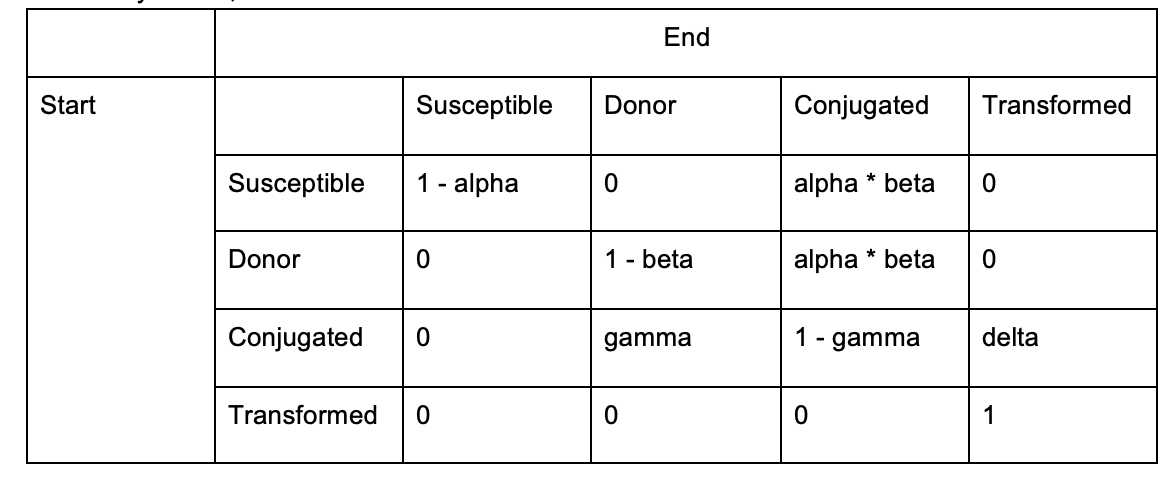In other words, the Markov Model can be mapped out as a collection of states with only intuitively selected paths of transition and its corresponding probability.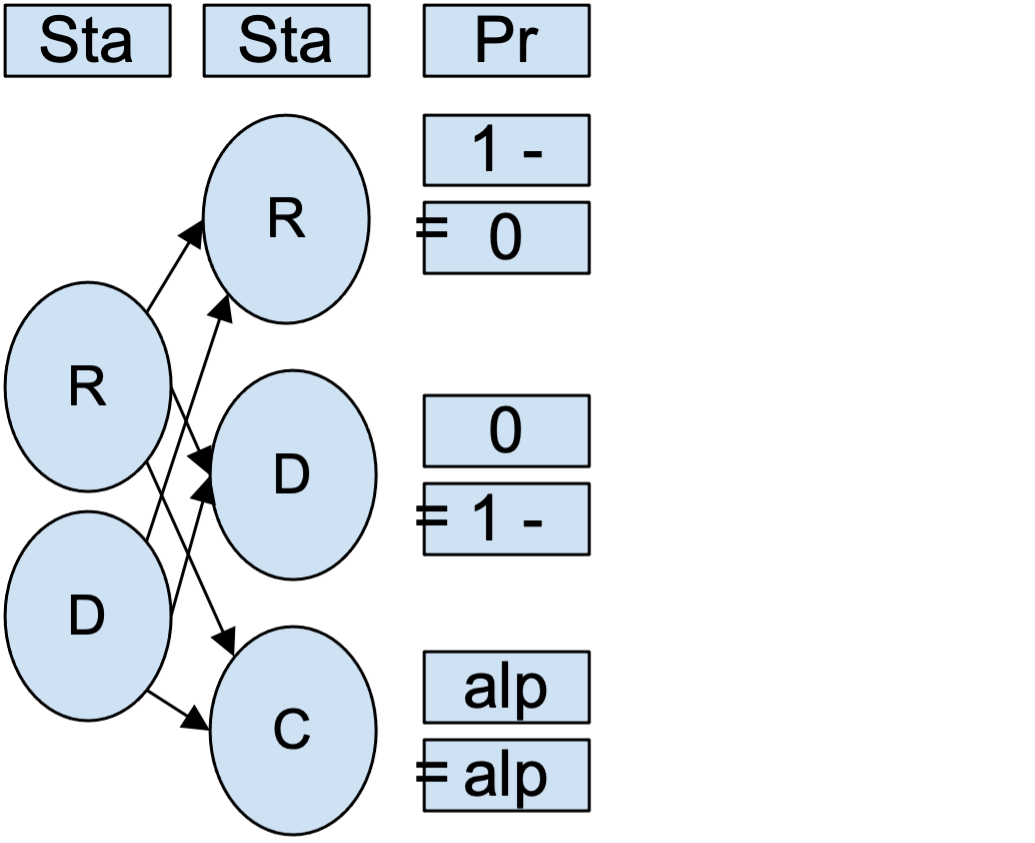The Markov Model, while simple and robust, required more agent-based variables corresponding to experimental conditions. Hence, the team implemented the End-point Method, to incorporate concepts of kinetics, resources, and OD600. The slider mechanism was preserved to ensure this model as a tool for biocontainment-related experiments, beyond the needs of Synterception.

## End-Point Method 

### Introduction

The End-point method is a derivation of plasmid rates of transfer and its relationship to cell growth in a liquid culture. In the beginning, the system of derivatives involved rate of transfer (Ѱ) and growth (𝛾), Donors (D), Recipients (R), Transconjugants (T), and Concentration of Resources (C). These rates of change reflect transitions in cell densities.

The method operates under 4 assumptions:
1. Conjugation occurs randomly and proportionally to plasmid and non-plasmid bearing cells
2. There is no plasmid loss in the system
3. Newly transformed cells become capable of donating plasmids
4. All clones grow at the same rate

### Approach

The approach for this model was to implement variations in rates of change in plasmid transfer according to different conditional parameters. In doing so, the plots sequentially become more complex but increasingly more accurate as growth and transfer are refined. Similar to the Markov Model, the equations were eventually applied to transition probabilities to simulate end-point steady-state using resource concentrations, OD600, and time.Above, the figure demonstrates the changes in cell densities with respect to time. The slider indicates the values used in the derived equations and produces results that preliminarily evaluate the occurrence of transconjugant cells.

Monod Functions advance the model under the assumption that the growth rate is a function of resource concentration, such that when the resources are depleted the Markov transitions (and transformation) ceases. The purpose of making this assumption is to isolate rates happening in the exponential phase as well as at half-saturation (Q).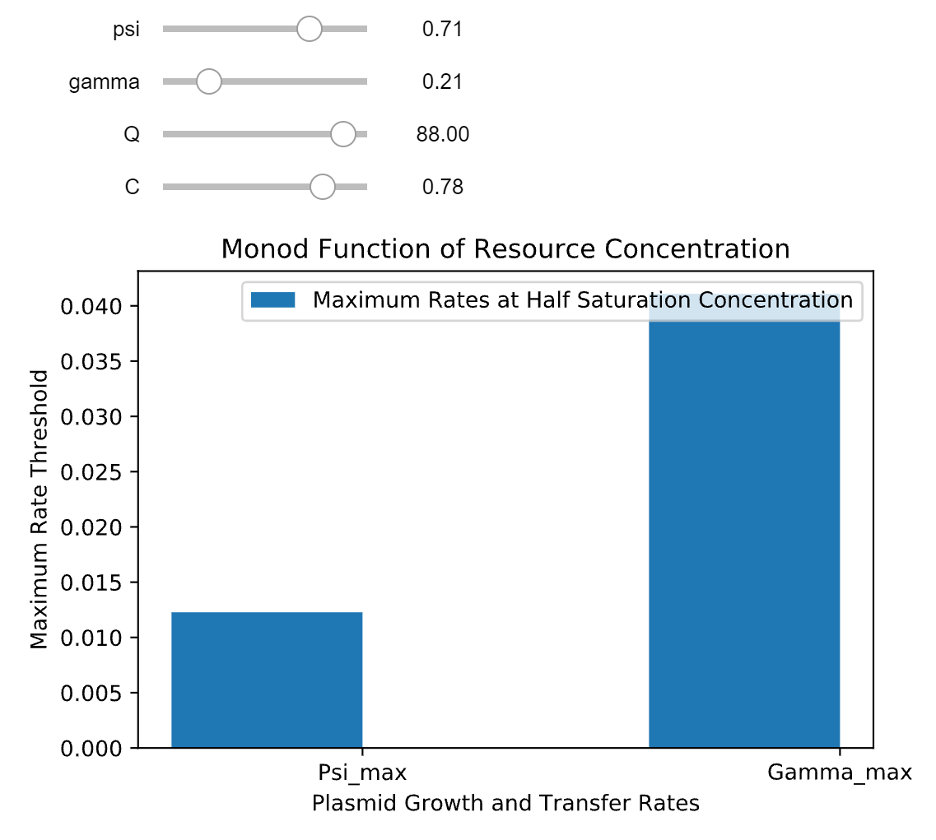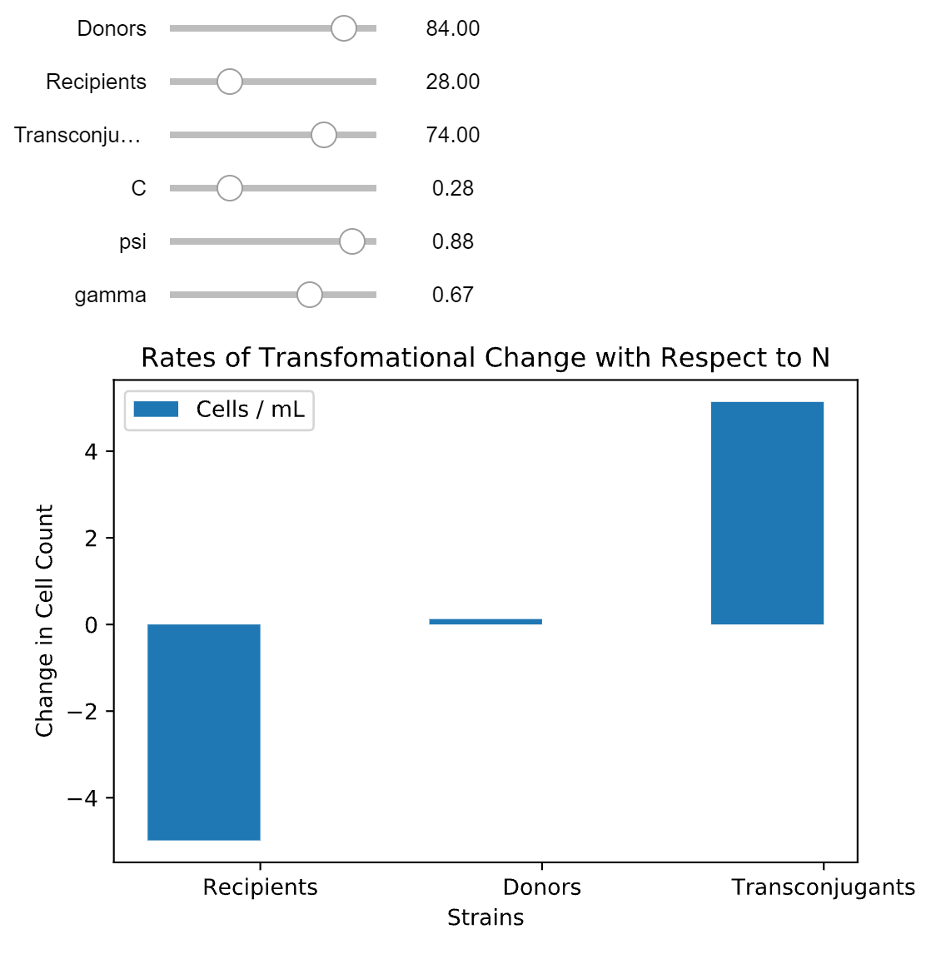With respect to the total number of cells, the model can be evolved to account for rates of transfer and growth in liquid culture.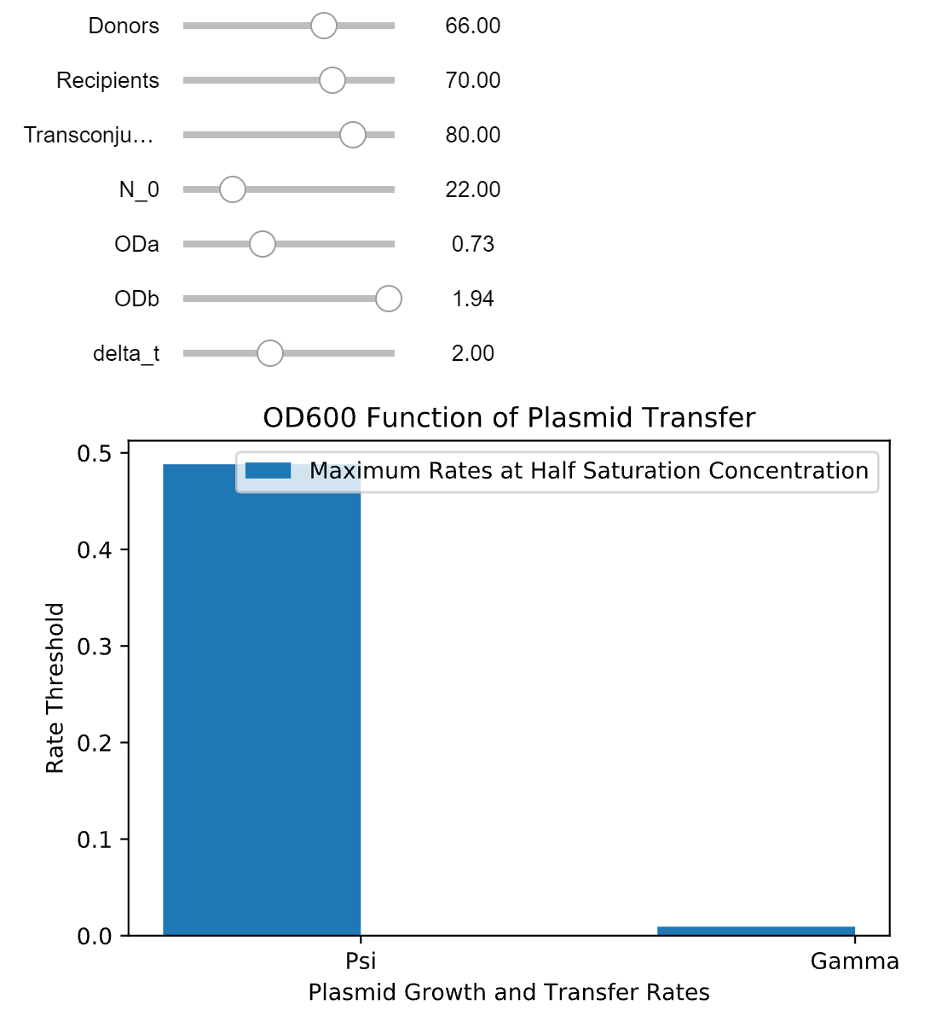Above, the figure demonstrates changes in Strains based on the additional relationship between variable rates of plasmid transfer and cell growth.Finally, the model below has developed into a final robust program that simulates the Markov steady-state of plasmid transfer in donor, susceptible (recipient), conjugated, and transformed (transconjugant) cell types. The probability matrix was adjusted to account for where cell growth and vector transfer, a function of OD600, would prevail similarly to the BMM. Then the initial state was converted to a slider to, once more, be a tool for modeling conjugative species.The figure above demonstrates an End-Point Markov Model. The plot is a result of a Python script initialized with the donor (pink), recipient (brown), transformed (blue), and one conjugated cell (green). Similar to the BMM, the program calculates the states over time, with agent-based conditions such as OD600, change in time from OD600 collection, along with the initial number of total cells in a liquid culture.

### Contribution

The End-Point Markov Model computation brings to Synterception. This model provides the tools and basis for understanding the kinetics and agents of end-point transformation via analysis of OD600, rates of plasmid transfer and cell growth, total cell density (in liquid culture), and time.

### Calculations and Notes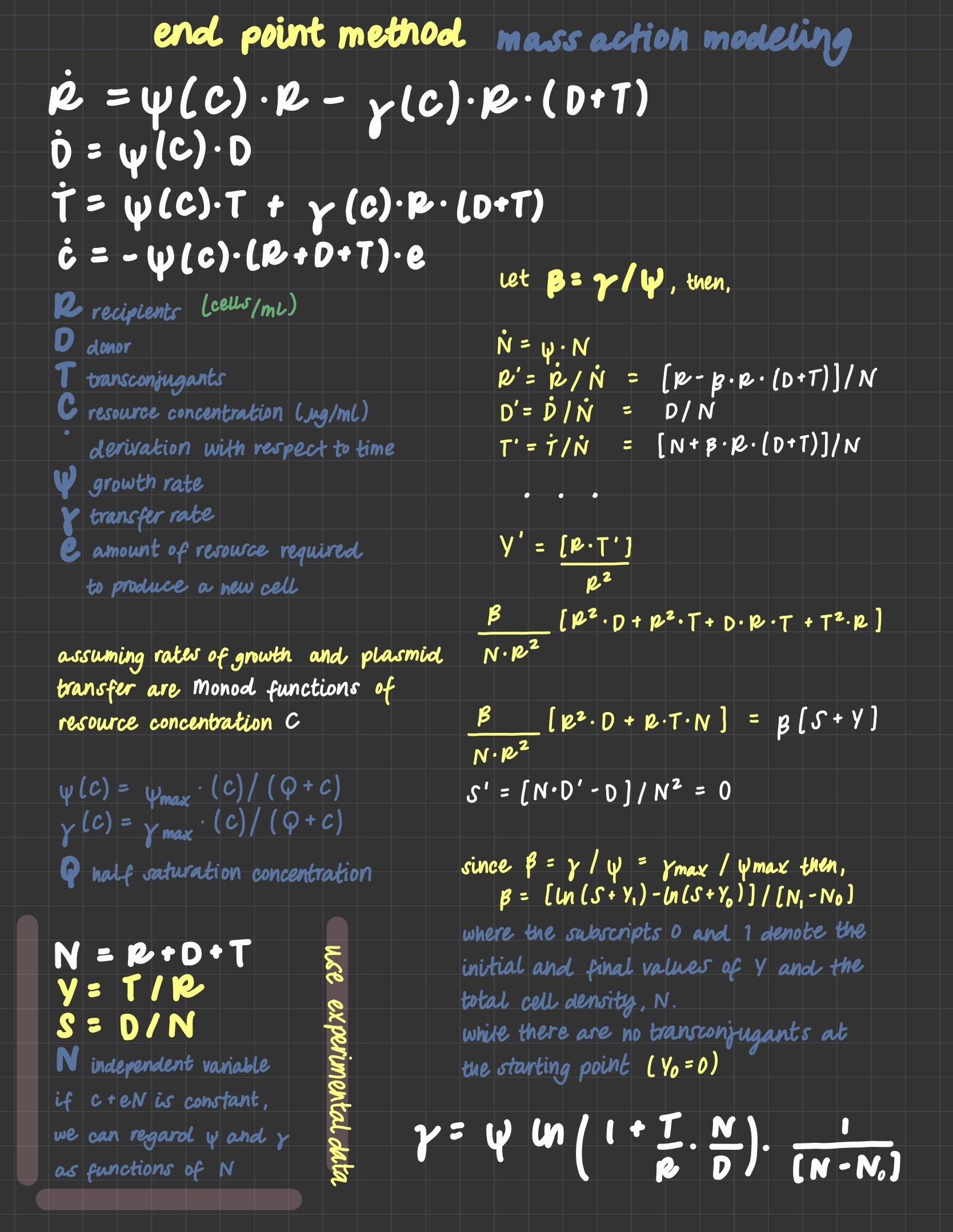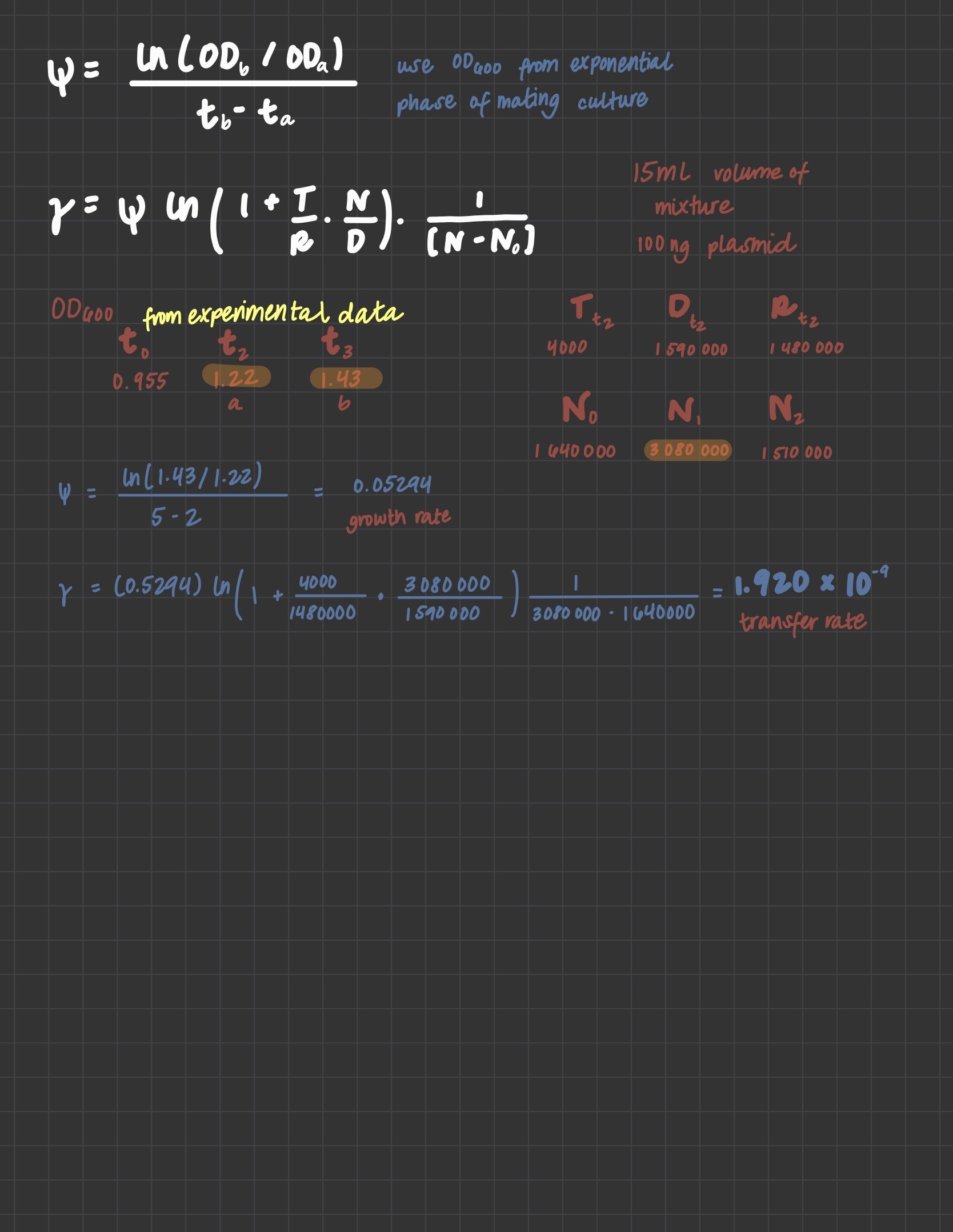Simonsen, L., Gordon, D. M., Stewart, F. M., & Levin, B. R. 1990. Estimating the rate of plasmid transfer: An end-point method. Journal of General Microbiology, 136: 11, 2319-2325. doi:10.1099/00221287-136-11-2319